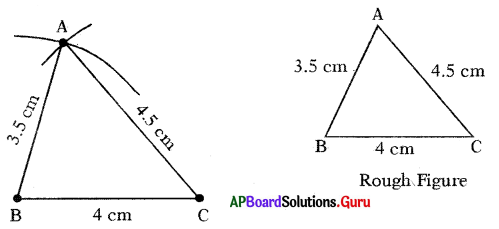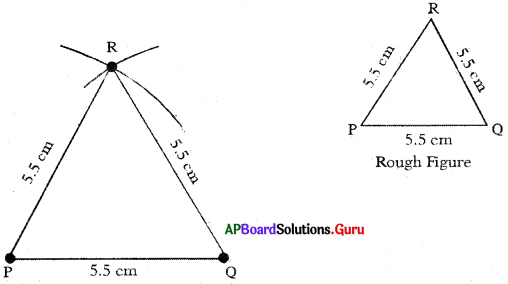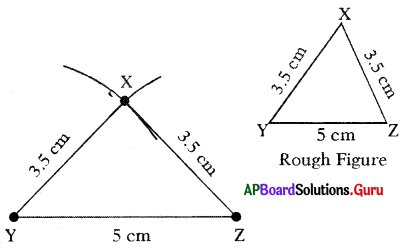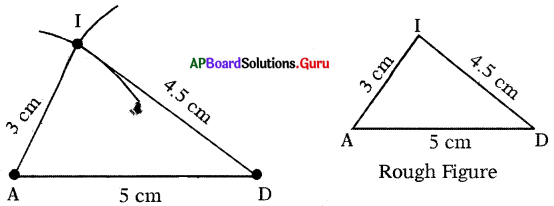# AP Board 7th Class Maths Solutions Chapter 10 Construction of Triangles Ex 10.1

SCERT AP 7th Class Maths Solutions Pdf Chapter 10 Construction of Triangles Ex 10.1 Textbook Exercise Questions and Answers.

## AP State Syllabus 7th Class Maths Solutions 10th Lesson Construction of Triangles Ex 10.1

Question 1.
Construct ∆ABC with measurements such that AB = 3.5 cm, BC = 4 cm and AC = 4.5 cm.
Given sides of ∆ABC are AB = 3.5 cm, BC = 4 cm and AC = 4.5 cm.Steps of Construction:

1. Draw a rough sketch of the triangle and label it with the given measurements.
2. Draw a line segment BC of length 4 cm.
3. Draw an arc with centre B and radius 3.5 cm.
4. Draw another arc with centre C and radius 4.5 cm to intersect the previous arc at A.
5. Join AB and AC.
Thus, required ∆ABC is constructed with the given measurements.Question 2.
Construct an equilateral triangle PQR with side PQ = 5.5 cm
Given sides of an equilateral triangle ∆PQR is PQ = 5.5 cm.
In an equilateral triangle all sides are equal in length.
∴ PQ = QR = PR = 5.5 cmSteps of Construction:

1. Draw a rough sketch of the triangle and label it with the given measurements.
2. Draw a line segment PQ of length 5.5 cm.
3. Draw an arc with centre P arid radius 5.5 cm.
4. Draw another arc with centre Q and the same radius (5.5 cm) to intersect the previous arc at R.
5. Join PR and QR.
Thus, required ∆PQR is constructed with the given measurements.

Question 3.
Construct ∆XYZ with measurements XY = 3.5 cm, YZ = 5 cm and ZX = 3.5 cm. Which type of triangle is this ? . ‘
Given sides of the ∆XYZ are XY = 3.5 cm, YZ 5 cm and ZX = 3.5 cm. In ∆XYZ two sides (XY = ZX = 3.5 cm) are equal.
So, ∆XYZ is an isosceles triangle.Steps of Construction:

1. Draw a rough sketch of the triangle and label it with the given measurements.
2. Draw a line segment YZ of length 5 cm.
3. Draw an arc with centre Y and radius 3.5 cm.
4. Draw another arc with centre Z and same radius (3.5 cm) to intersect the previous arc at X.
5. Join XY and XZ.
Thus, required ∆XYZ is constructed with the given measurements.Question 4.
Construct a triangle with measurements of 5 cm, 3 cm and 4.5 cm.